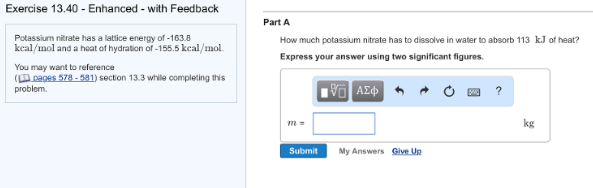# Problem: Potassium nitrate has a lattice energy of -163.8 kcal/mol and a heat of hydration of -155.5 kcal/mol. Part AHow much potassium nitrate has to dissolve in water to absorb 113 kJ of heat? Express your answer using two significant figures.

###### FREE Expert Solution
80% (312 ratings)###### Problem Details

Potassium nitrate has a lattice energy of -163.8 kcal/mol and a heat of hydration of -155.5 kcal/mol.

Part A

How much potassium nitrate has to dissolve in water to absorb 113 kJ of heat?